• +91 9971497814
• info@interviewmaterial.com

# RD Chapter 13- Complex Numbers Ex-13.3 Interview Questions Answers

### Related Subjects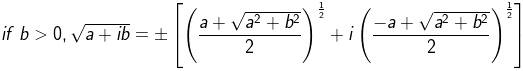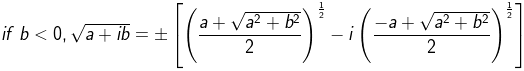(i) – 5 + 12i

Given:

– 5 + 12i

We know, Z = a + ib

So, √(a + ib) = √(-5+12i)

Here, b > 0

Let us simplify now,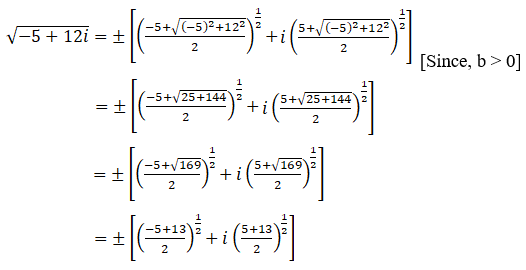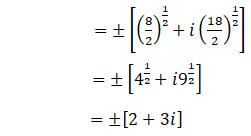Square root of (– 5 +12i) is ±[2 + 3i]

(ii) -7 – 24i

Given:

-7 – 24i

We know, Z = -7 – 24i

So, √(a + ib) = √(-7 – 24i)

Here, b < 0

Let us simplify now,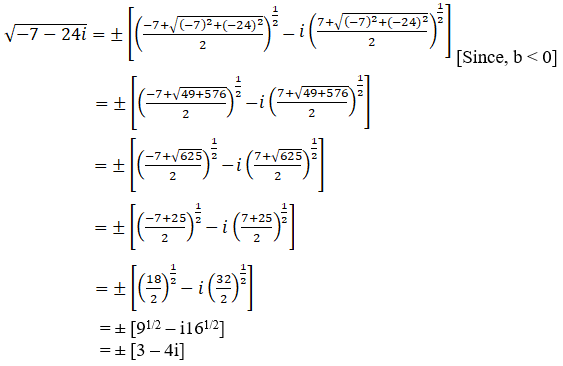Square root of (-7 –24i) is ± [3 – 4i]

(iii) 1 – i

Given:

1 – i

We know, Z = (1 – i)

So, √(a + ib) = √(1 – i)

Here, b < 0

Let us simplify now,

Square root of (1 –i) is ± [(√(√2+1)/2) – i (√(√2-1)/2)]

(iv) -8 -6i

Given:

-8 -6i

We know, Z = -8 -6i

So, √(a + ib) = -8 -6i

Here, b < 0

Let us simplify now,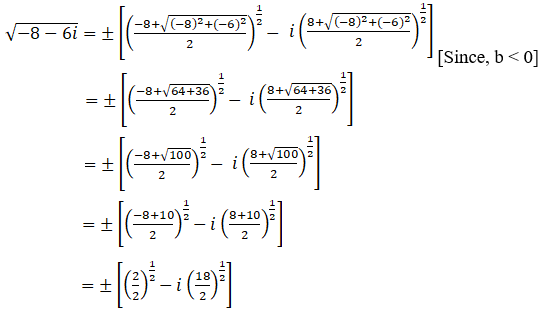= [11/2 –i 91/2]

= ± [1 – 3i]

Square root of (-8-6i) is ± [1 – 3i]

(v) 8 – 15i

Given:

8 – 15i

We know, Z = 8 – 15i

So, √(a + ib) = 8 – 15i

Here, b < 0

Let us simplify now,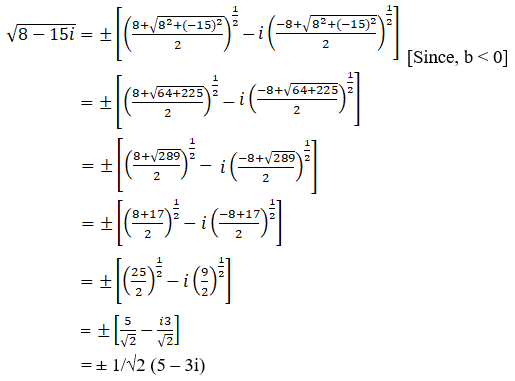Square root of (8 –15i) is ± 1/√2 (5 – 3i)

(vi) -11 – 60√-1

Given:

-11 – 60√-1

We know, Z = -11 – 60√-1

So, √(a + ib) = -11 – 60√-1

= -11 – 60i

Here, b < 0

Let us simplify now,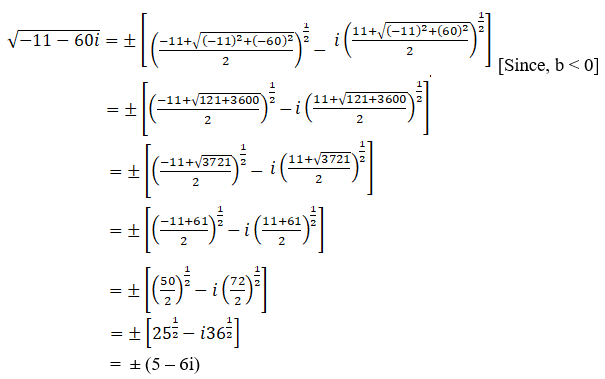Square root of (-11 –60√-1) is ± (5 – 6i)

(vii) 1 + 4√-3

Given:

1 + 4√-3

We know, Z = 1 + 4√-3

So, √(a + ib) = 1 + 4√-3

= 1 + 4(√3) (√-1)

= 1 + 4√3i

Here, b > 0

Let us simplify now,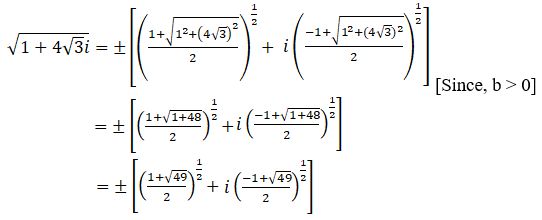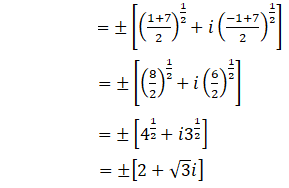Square root of (1 + 4√-3) is ± (2 + √3i)

(viii) 4i

Given:

4i

We know, Z = 4i

So, √(a + ib) = 4i

Here, b > 0

Let us simplify now,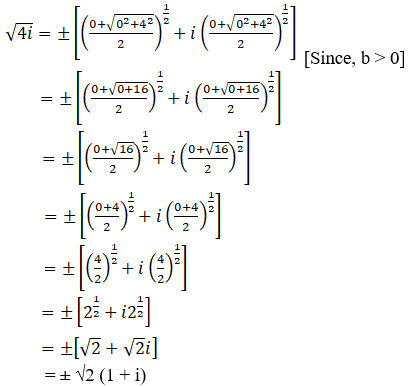Square root of 4iis ± √2 (1 + i)

(ix) –i

Given:

-i

We know, Z = -i

So, √(a + ib) = -i

Here, b < 0

Let us simplify now,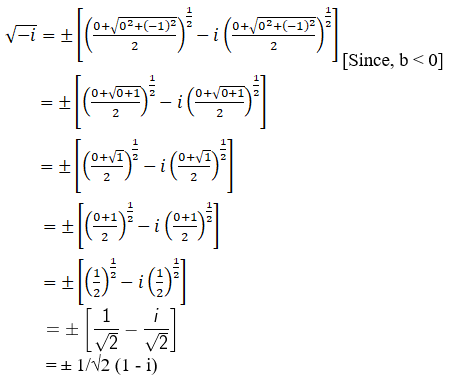Square root of –iis ± 1/√2 (1 – i)

Todays Deals### RD Chapter 13- Complex Numbers Ex-13.3 Contributorskrishan

Name:
Email:

# Latest News# 9000 interview questions in different categories